## Chapter 9: Search Trees I

We study several alternative data structures based on trees for realizing ordered dictionary. The fundamental operations in an ordered dictionary ADT are:
• find(k) - Return the position of an item with key k, and return a null position if no such item exists.
• findAll(k) - Return an iterator of positions for all items with key k.
• insertItem(k,e) - Inserts an item with element e and key k.
• removeElement(k) - Remove an item with key k. An error condition occurs if there is no such item.
• removeAllElements(k) - Remove all items with key equal to k.
• closestBefore(k)- Return a position of an item with the largest key less than or equal to k.
• closestAfter(k) - Return a position of an item with the smallest key greater than or equal to k.

### 9.1 Binary Search Trees (reminder)

• A binary search tree is a binary tree storing keys (or key-element pairs) at its internal nodes and satisfying the following property:
• Let u, v, and w be three nodes such that u is in the left subtree of v and w is in the right subtree of v.
We have key(u) ≤ key(v) ≤ key(w)
• External nodes do not store items
• Inorder traversal visits the keys of its dictionary in non-decreasing order.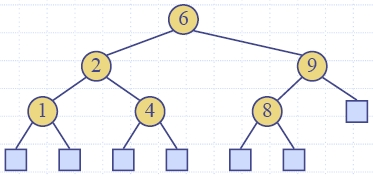9.1.1 Searching

• To search for a key k, we trace a downward path starting at the root
• The next node visited depends on the outcome of the comparison of k with the key of the current node
• If we reach a leaf, the key is not found and we return a null position
 Algorithm find (k, v)     if T.isExternal (v)         return Position(null)    if k < key(v)         return find(k, T.leftChild(v))    else if k = key(v)         return Position(v)    else { k > key(v) } return find(k, T.rightChild(v))
• Example: find(4)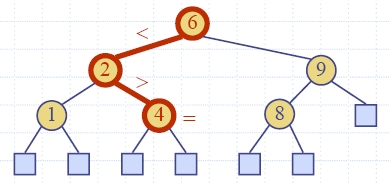Analysis of Binary Tree Searching
• Function find(k) on dictionary D runs O(h) time, where h is the height of the binary search tree T used to implement D.

#### 9.1.2 Update Operations

Insertion
• To perform operation insertItem(k, e), we search for key k
• Assume k is not already in the tree, and let w be the leaf reached by the search
• We insert k at node w and expand w into an internal node
• Example: insert 5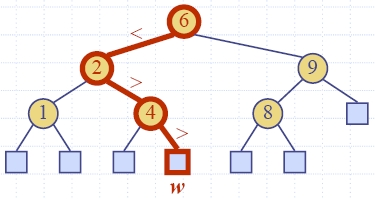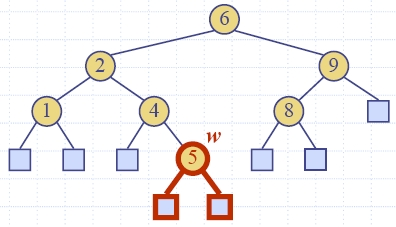Removal
• To perform operation removeElement(k), we search for key k
• Assume key k is in the tree, and let let v be the node storing k
• If node v has a leaf child w, we remove v and w from the tree with operation removeAboveExternal(w)
• Example: remove 4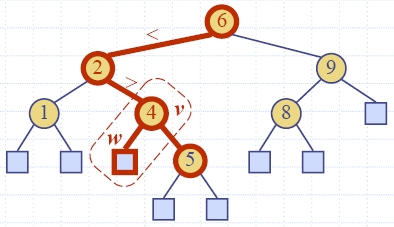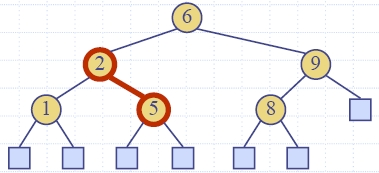• We consider the case where the key k to be removed is stored at a node v whose children are both internal
• we find the internal node w that follows v in an inorder traversal
• we copy key(w) into node v
• we remove node w and its left child z (which must be a leaf) by means of operation removeAboveExternal(z)
• Example: remove 3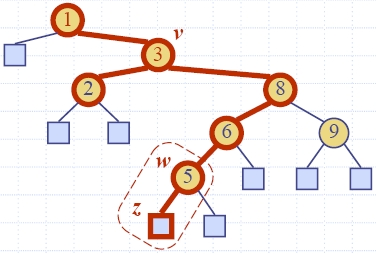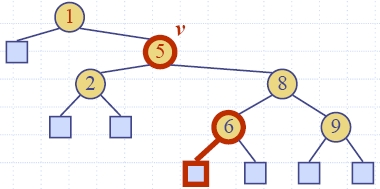Best-case versus Worst-case

• Consider a dictionary with n items implemented by means of a binary search tree of height h
• the space used is O(n)
• methods findElement(), insertItem() and removeElement() take O(h) time
• The height is O(n) in the worst case and O(log n) in the best case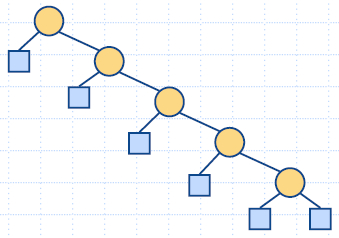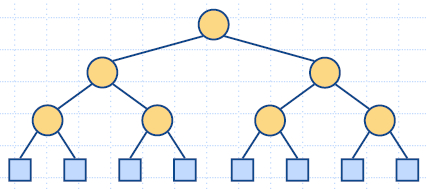#### 9.1.3 C++ Implementation

A Binary Search Tree in C++
html-9.2 (Position)
html-9.3 (BST1)
html-9.4 (BST2)
html-9.5 (BST3)
html-9.6 (BST4)

except.h  -   LBTree.h   -   BSTree.cpp

### 9.2 AVL Trees

• Height-Balance Property: For every internal node v of T the heights of the children of v can differ by at most 1.
• An AVL Tree is a binary search tree with Height-Balance Property.
• Adel'son-Vel'skii and Landis (Адельсон-Вельский, Ландис - 1968)
• Proposition: The height of an AVL tree storing n keys is O(log n).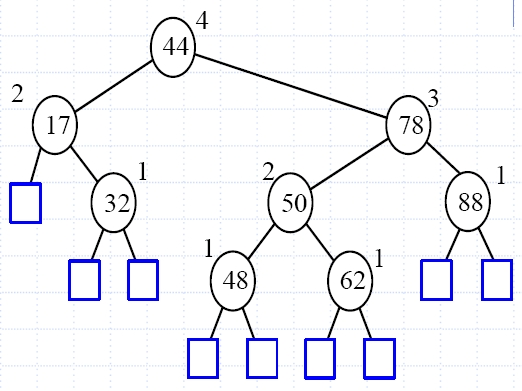Insertion in an AVL Tree
• expandExternal(w)
• Let z be the first node we encounter in going up from w toward the root of T, such that z is unbalanced.
• Let y denote the child of z with higher height.
• Let x be the child of y with higher height.
Trinode Restructuring after an Insertion
• Let (a, b, c) be an inorder listing of x, y, z, perform the rotations needed to make b the topmost node of the three.
• Example: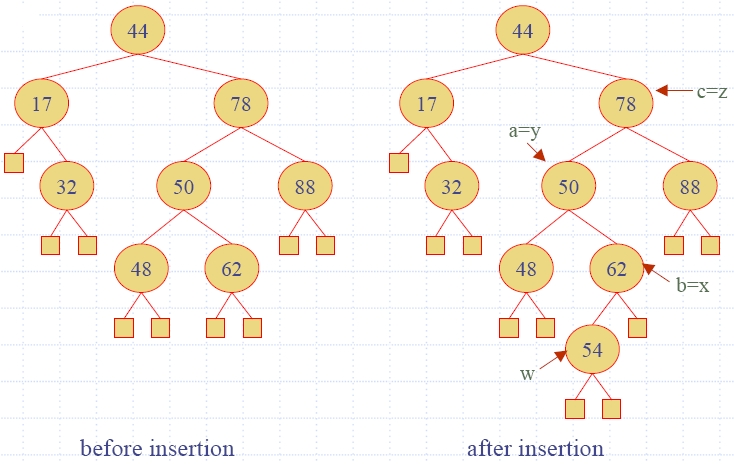• There are 4 possible ways of mapping (x, y, z) to (a,b,c).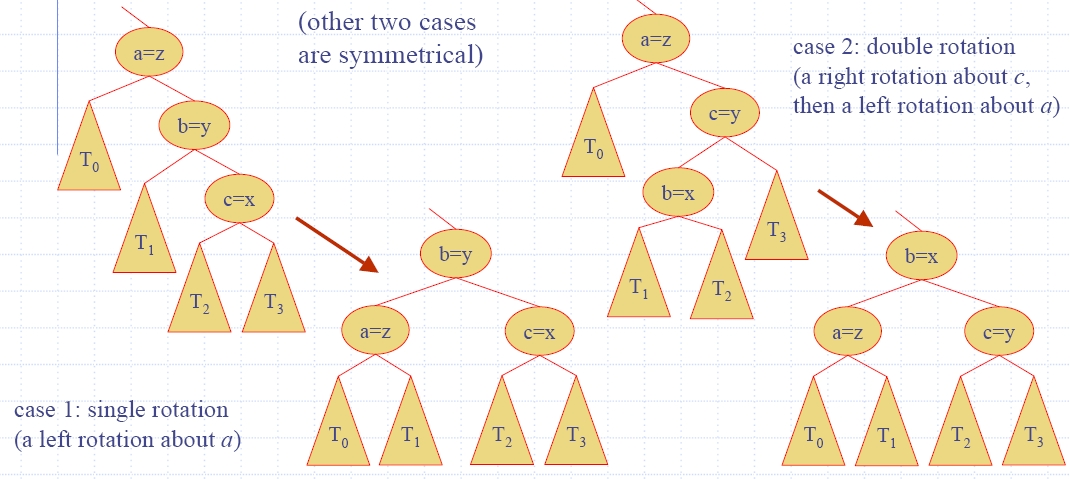• Example: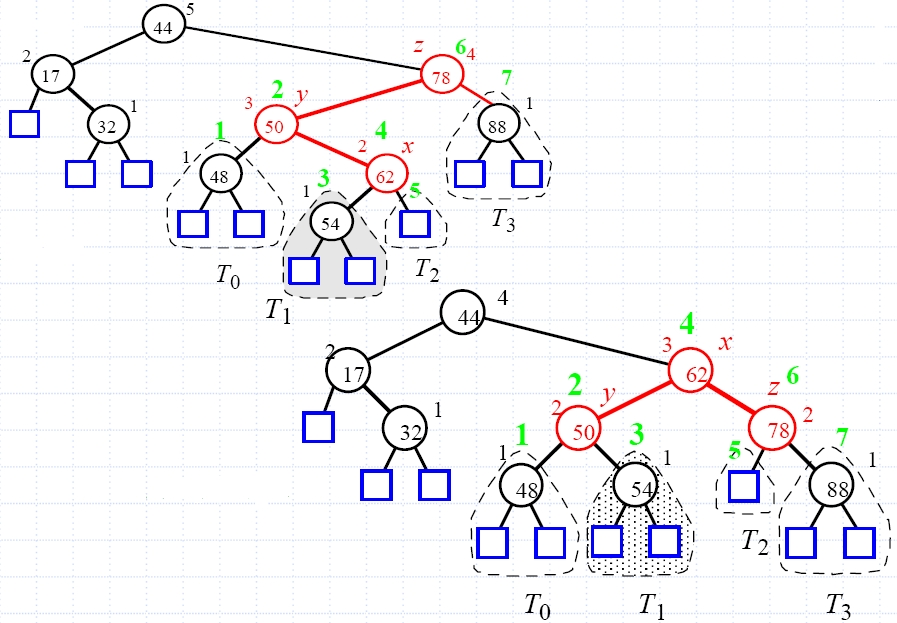Removal in an AVL Tree
• removeElement(k)
• Removal begins as in a binary search tree, which means the node removed will become an empty external node.
• Its parent, w, may cause an imbalance.
• Example: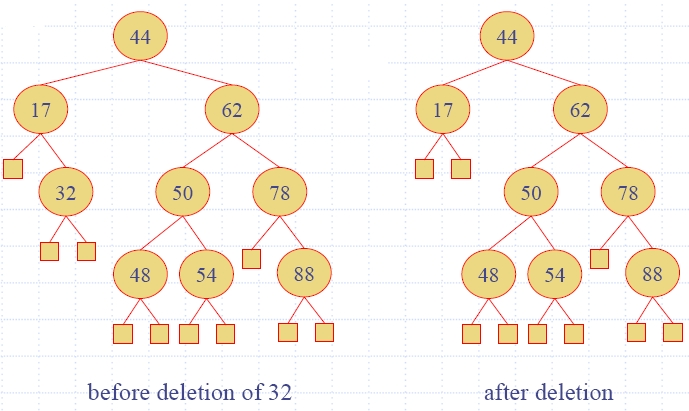Rebalancing after a Removal
• Let z be the first unbalanced node encountered while traveling up the tree from w.
• Also, let y be the child of z with the larger height, and
• let x be the child of y with the larger height.
• We perform restructure(x) to restore balance at z.
• As this restructuring may upset the balance of another node higher in the tree, we must continue checking for balance until the root of T is reached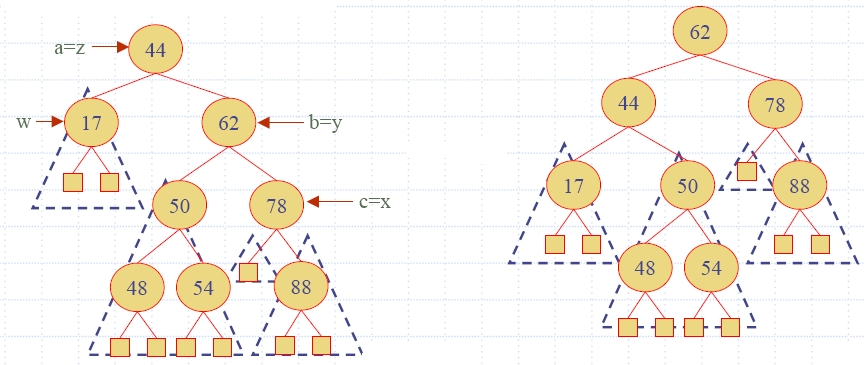Running Times for AVL Trees

• a single restructure is O(1)
• using a linked-structure binary tree
• find is O(log n)
• height of tree is O(log n), no restructures needed
• insert is O(log n)
• initial find is O(log n)
• Restructuring up the tree, maintaining heights is O(log n)
• remove is O(log n)
• initial find is O(log n)
• Restructuring up the tree, maintaining heights is O(log n)

#### 9.2.2 C++ Implementation

html-9.8 (AVL Item)
html-9.9 (AVL Tree 2)
html-9.10 (AVL Tree 1)